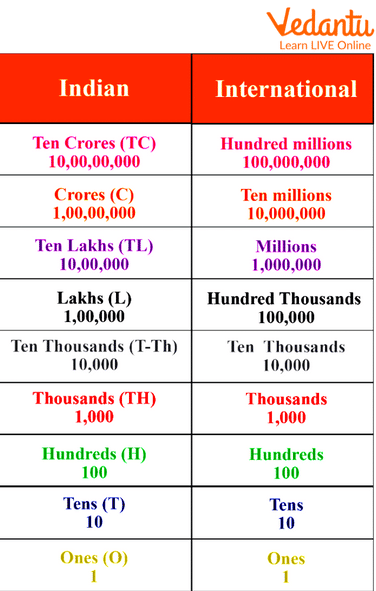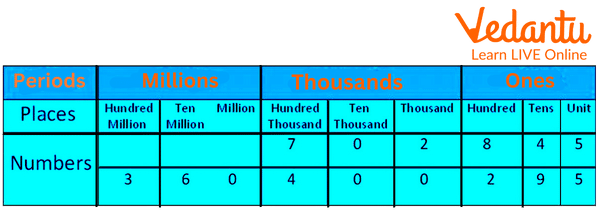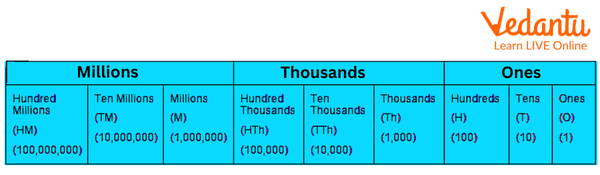Courses
Courses for Kids
Free study material
Free LIVE classes
More

# International Number System## Introduction to Number System

The international numeracy system, also known as the international numeracy system, is followed and used in many countries today. While counting numbers was previously done with stones, blocks, and sticks, the numeral system was introduced to make the process more efficient. The numeral system is of two kinds: the Indian Numeral System and, therefore, the International Numeral System. The Indian numeral system is widely utilised in India, while the International numeral system is used globally. Let’s learn more about the international number system and solve a few examples for better understanding.

### Rules of International Numeration System

In the international number system, the location of the separators (commas) is extremely important. These separators are done consistently with their place values and the periods of any number. Here is the basic rule in the international numeral system:

• The periods aren’t written in the singular form. For instance, \[456,876\] is usually written as "four hundred fifty-six thousand, eight hundred seventy-six”. We don’t add an "s" to a thousand or a hundred.

• The word "and" isn’t used before tens and ones. For instance, \[3535\] is usually read as three thousand five hundred thirty-five. We don’t read it as three thousand and five hundred thirty-five.

• Commas aren’t used when we write the number in an expanded form. For instance, \[3535\] is usually written as three thousand five hundred thirty-five. We don’t write it as "three thousand, five hundred, thirty-five."

## What is the International System of Numeration?

The majority of countries around the world uses the International System of Units of Numeracy. In this system, too, variety is split up into groups or periods. We start from the acute right digit of the number to form the groups. The group's names are known as ones, thousands, millions, and billions.

1. The ones, in turn, are divided into hundreds, tens, and units.

2. The second of the subsequent three digits on the left of the group of ones form the group of thousands, which is further split up into thousands, ten thousand, and hundred thousand.

3. The third group of the subsequent three digits on the left of the group of thousands is the group of millions.

4. The three digits on the left of the group of millions form a gaggle of billions, split into billion, ten billion, and 100 billion.

## Numeration Chart

Indian Numeration: The place values of the digits in the Indian numeral system are Ones, Tens, Hundreds, Thousands, Ten Thousand, Lakhs, Ten Lakhs, Crores, and so on.

International Numeration: In the international numeral system, the place values of digits are arranged in the following order: ones, tens, hundreds, thousands, ten thousand, hundred thousand, millions, ten million, and so on.Numeration Chart

## International Numeration System Table

The international number system uses a division of numbers into groups, or "periods," to represent numbers. We are able to properly read enormous numbers thanks to these groupings or periods. Similar groups or periods exist in the the international numbering system, but the location of the commas is different. The ones, thousands, millions, etc., are the periods in the international numbering system. These periods are further broken down into groups. To understand the period system in the international numbering system, look at the table below.International Numeration Table

## International Numeration System Example

Example 1: Rewrite the amount 1436915 in the International numeral system, using commas. How will you read this number consistent with both systems?

Solution:

In the International numeral system, we start from the right side and insert commas after every three digits. We divide the amount into periods and read the period separately.

Standard International numeral form: \[1,436,915\]

Number name or expanded form: One million four hundred thirty-six thousand nine hundred fifteen.

## International Numeration System Chart

As mentioned before, the international numeration system is divided into periods such as ones, thousands, millions, etc. Each of those periods is further divided into groups according to the place value of a number. Here is the demarcation of the periods:

• 1: The one period is re-divided into further groups of 3, i.e., ones, tens, and hundreds. As a result, the number's first three digits fall within this period.

• 1000: The thousand period is re-divided into further groups of 3, i.e., thousands, tens of thousands, and hundred thousand. As a result, the three digits following the first period of any number fall under this period.

• Million Period: The million period is re-divided into further groups of 3, i.e., million, ten-million, and hundred-million. Hence, the subsequent 3 digits after the thousand periods of any number come under this period.

• Billion Period: The billion period is grouped into three, i.e., billion, ten-billion, and hundred-billion. Hence, the subsequent 3 digits after the million periods of any number come under this period.

Let us look at an image showcasing the period and the place value of the international number system.International Numeration Chart

While writing numbers within the international number system, separators (commas) are used after every period while representing a variety in its standard numeral form. Whereas for reading, the digits within the same period are read together, and therefore, the name of the period (except the ones) is read along with them. Allow us to look at an example: 18357254. This number is written as 18,357,254, and in words, it will be written as "eighteen million, three hundred fifty-seven thousand, two hundred fifty-four."

## Solved Questions

1: Rewrite the number 2977213 by placing the commas as per the international number system.

Solution:

• Starting from the proper side of the number, the primary comma is inserted after 3 digits of the one's period.

• The second comma is inserted after the subsequent 3 digits i.e. the thousands period.

• The third comma is inserted after the millions period.

Therefore, the given number \[2977213\] will be written as \[2,977,213.\]

2: Write the expanded form within the international number system. 100 twenty-two million one hundred twenty-two thousand one hundred forty-three.

Solution: The expanded sort of One hundred twenty-two million one hundred twenty-two thousand one hundred forty-three can be written as: \[122,122,143.\]

## Summary

In this chapter, we have studied the number system. The international number system is the rule or set of reading numbers that are valid worldwide. We have also covered the topic of the rule of international law and solved various examples.

Last updated date: 24th May 2023
Total views: 43.5k
Views today: 0.42k

## FAQs on International Number System

1. What is meant by place value?

Any digit in a number has a place value in mathematics determined by its position. For instance, the place value of 4 in 345 is 40 because it is in the tenth position.

2. What do you mean by face value?

The value of a digit in a number is its face value. For instance, if the number 567 is a number, the face value of the digit 6 is only 6.

3. In the international numbering system, how many zeros make up one billion?

1 billion equals 1,000,000 in the international numbering system. Therefore, a billion has six zeros.